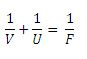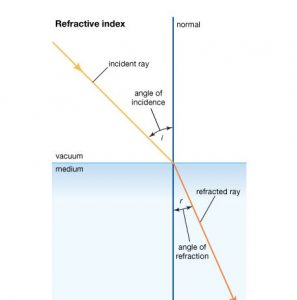Physic Tutorials

# Notes On Optics : Light Waves For O’Level

Notes on optics : light waves

Transmission of light

Light can travel through a solid, liquid and gas or vacuum. Therefore, it does not need a maternal medium for its transmission.

Types of object/substance

1          Opaque object: These are objects that do not allow light to pass through them, e. g wood, stone, table, wall, etc.

2         Transparent objects: Transparent objects are those which allow some light energy to pass through them, e. g plane glass, clean and clear water, etc.

3          Translucent object: This allow some light to pass through them, but object cannot be seen clearly through them, e. g some window panes, cloth.

Rectilinear Propagation of Light

Rectilinear propagation of light is the phenomenon of light travelling in a straight line. The phenomenon can be demonstrated by using a candle flame, a string and three pieces of cardboard.

The pinhole camera

This operates on the principle that light travels in a straight line. It consists of a light-tight box, one end of which has a small hole made with a pin or needle point. If the pinhole is small, the image is bright (sharp) but when it is large a brighter but blurred image is obtained. A wide hole will produce several images that overlap that are seen as single blurred image. When the object distance is far from the pinhole, it produces a invented but diminished image. Also, when the distance between the pinhole and the screen is increased, the image is enlarged but less bright.

Magnification produced by a pinhole camera = image distance / object distance or image height/ object height

Laws of Reflection

• The angle of incidence is equal the angle of reflection
• The incident ray, the reflected ray and the normal at the point of incidence all lie in the same plane.

Formation of image by a plane mirror

The images formed by a plane mirror has the following characteristics

• It is erect
• It is far behind the mirror as the object is in front
• It is virtual
• It has the same size as the object
• Laterally inverted

Image formed by inclined mirror

When two mirrors are inclined at a certain angle to each other, and an object is placed in front of them, the number of images seen is given by

N =( 360/θ) – 1

Where the angle of inclination and N is the number of images formed

Uses of the plane mirror

• As looking glass
• In the periscope of two plane mirrors inclined at and fixed facing each other
• Plane mirror can be used as kaleidoscope. It consists of three plane mirrors inclined at   to each other and a piece of ground glass set at the bottom.

Curved or spherical mirror

Curved mirror are produced by cutting out a part of a spherical shell: it is called concave mirror when light is reflected from the inside and convex mirror when light is reflected from the outside.

A concave mirror can form invented, real and magnified image of the object or real, inverted and diminished  image or erect, virtual and magnified image depending on where the object is located.

• When the object is beyond C – Nature of image is real, inverted and diminished
• When the object is at C – Nature of image is real, inverted and same size
• When the object is between F and C – Nature of image is real, inverted and magnified
• When the object is at f – Nature of image is real, inverted, but formed at infinity
• When the object is between p and f – Nature of image is virtual, erect and magnified

C is the centre of curvature

F is the principal focus

p is the pole

Use of the curved mirror

• Convex mirror is used as car driving mirrors because they form upright image and also has a wild field of view.
• Convex mirror is also use in a super market to see round corners.

Concave mirrors

• Used as shaving mirror.
• Used as the distance
• Used in focusing starts for astronomical studies when used in the astronomical telescope.

Mirror formula can also be used to find the nature of the image formed by concave and convex mirrorWhere V = image distance U = object distance F = focal length, F = r/2

Example

An object 8cm high placed 30cm from a concave mirror of radius of curvature 10cm. Calculate the (i) image distance (ii) image height

solution

U = 30cm  f = 20/2 = 10cm

using the mirror formula

1/f = 1/u + 1/v

1/5 = 1/30 + 1/v

1/v = 1/5 – 1/30

1/v = 6/30

v = 5 cm

image distance = 5 cm

image distance/object distance = image height / object height

5/30 = x/8

x = 40 / 30

x = 1.3 cm

image height = 1.3 cm

Refractive index

Refractive index, also called index of refraction, measure of the bending of a ray of light when passing from one medium into another. If i is the angle of incidence of a ray in vacuum (angle between the incoming ray and the perpendicular to the surface of a medium, called the normal) and r is the angle of refraction (angle between the ray in the medium and the normal), the refractive index n is defined as the ratio of the sine of the angle of incidence to the sine of the angle of refraction; i.e., n = sin i / sin r. Refractive index is also equal to the velocity of light c of a given wavelength in empty space divided by its velocity v in a substance, or n = c/v. The speed of light is not constant as it moves from medium to medium. When light enters a denser medium (like from air to glass) the speed and wavelength of the light wave decrease while the frequency stays the same. How much light slows down depends on the new medium’s index of refraction, n. (The speed of light in a medium with index n is c/n.)UTME past questions and solutions on optics

### Bolarinwa Olajire

A lecturer, Educationist, PhD student at FUNAAB, and a Blogger.